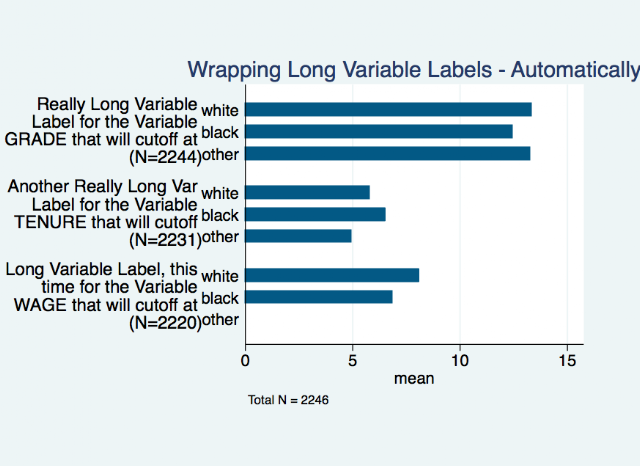While using -statplot- in the real world, we came across a situation where we needed to place the N's for sub-groups in certain value or variable labels.
For these figures, the N's change over sub-datasets and when the survey data is updated with each wave, so actually writing something like "(N = 100)" into each value or variable label or graph title is repetitive.  These figures are a heavy on the information side (they'd surely be an easy target for junkcharts for many reasons), but the real version of these figures use less N's than the examples below and they are made to mirror the output produced by Ian Watson's -tabout- (from SSC).
Here's a strategy to add some N's to graphs automatically & wrap these labels with N's.  This example follows from the examples presented in earlier posts about -statplot- here and here.
***********************************!Create Data Example
sysuse nlsw88, clear
**varlabels**
lab var grade "Really Long Variable Label for the Variable GRADE that will cutoff at 80 chars"
lab var tenure "Another Really Long Var Label for the Variable TENURE that will cutoff at 80 chars"
lab var wage "Long Variable Label, this time for the Variable WAGE that will cutoff at 80 chars"
replace wage = . if race==3
***********************************!Create Data Example

***********************!beginFig14
loc ll 25 //sets the length of the labels
loc j = 1
foreach u of local vars {
**calc N**
qui count if !mi(`u')
loc nn `r(N)'
di "`j'"
loc len = length("`:var l `u''")
if `len' > `ll' {
loc pieces "`=round(`len'/`ll')'"
forval p = 1/`pieces' {
loc p`p' : piece `p' `ll' of "`:var l `u''", nobreak
loc relabeling`j' `" `relabeling`j''  `"`p`p'' "'   "'
}
}
loc relabeling `" `relabeling'  `j'`"`relabeling`j'' "(N=`nn')" "' "'
loc `j++'
}
di "`relabeling'"
loc totalN = _N
****
statplot `vars',  ///
name(g2, replace)  over(race) graphregion(margin(vlarge)) ///
varopts( relabel( `relabeling' )) ///
title("Wrapping Long Variable Labels - Automatically") ///
note(Total N = `totalN')
graph export "fig14.png", as(png) replace
***********************!endFig14Fig 14

The next section does the same thing for value labels:
**value labels**
lab def racelbl 1 "White:  Really Long label for Race goes here" ///
2 "Black:  Another really long label " ///
3 "Other:  Another really long label", modify
lab val race racelbl
***********************!beginFig15
loc ll 20 //sets the length of the val labels
loc j = 1
foreach u in race {
loc z `"`:value label `u''"'
di "`z'"
**detect levels of each var**
levelsof `u', loc(`u'levels)
foreach uu in ``u'levels' {
**calc N**
qui count if `u'==`uu' & !mi(`u')
loc nn `r(N)'
loc len = length("`:label   `z' `uu' '")
di "`len'"
if `len' > `ll' {
loc pieces "`=round(`len'/`ll')'"
forval p = 1/`pieces' {
loc p`p' : piece `p' `ll' of "`:label  `z' `uu' '", nobreak
loc relabeling`j'`uu' `" `relabeling`j'`uu''   `"`p`p'' "'   "'
} //pieces
} //if
loc relabelinglabels `" `relabelinglabels'  `j' `"`relabeling`j'`uu'' "(N=`nn')" "'   "'
loc `j++'
} //levelsof
} //foreach
di "`relabelinglabels'"
loc totalN = _N
****
name(g2, replace) tit("Long VALUE Labels") ///
over(race, relabel( `relabelinglabels' ) ///
label(labsize(vsmall) labgap(2))) ///
varopts( relabel( `relabeling' ))  ///
note(Total N = `totalN')
graph export "fig15.png", as(png) replace
***********************!endFig15
*****************************************Fig 15
The -tabout- counterpart to this graph might look like:
********
tabout race using "\$table1.xls", replace sum ///
c(mean grade mean tenure mean wage n wage) lines(double) ///
npos(lab) nlab( (n=#) )
*******

Here's the do-file for this code.